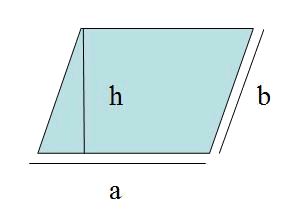# The rhomboidThe area of the romboide is: $$A=a \cdot h$$$Its perimeter is: $$P=2 \cdot (a+b)$$$

Calculate the area of a rhomboid with $$a=5 \ cm$$ and of height $$3 \ cm$$

$$A=3 \ cm \cdot 5 \ cm=15 \ cm^2$$\$

And so, the area of the romboide is: $$15 \ cm^2$$.